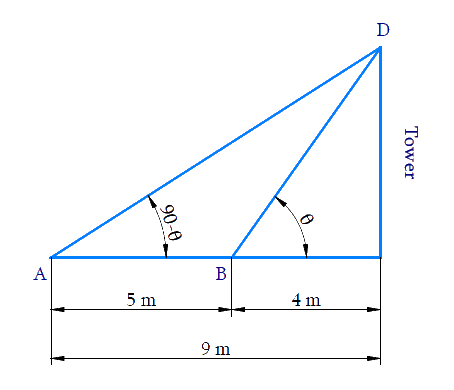# Ex.9.1 Q16 Some Applications of Trigonometry Solution - NCERT Maths Class 10

Go back to  'Ex.9.1'

## Question

The angles of elevation of the top of a tower from two points at a distance of $$4\,\rm{m}$$  and $$9\,\rm{m}$$  from the base of the tower and in the same straight line with it are complementary. Prove that the height of the tower is $$6\,\rm{m.}$$

Video Solution
Some Applications Of Trigonometry
Ex 9.1 | Question 16

## Text Solution

What is Known?

Angle of elevation of the top of a tower from two points at a distance of $$4\,\rm{m}$$ and $$9\,\rm{m}$$ from the base of the tower are complimentary.

What is Unknown?

To prove height of the tower is $$6\,\rm{m.}$$Reasoning:

Let the height of the tower as $$CD$$. B is a point $$4\rm{m}$$ away from the base $$C$$ of the tower and $$A$$ is a point $$5\rm{m}$$ away from the point $$B$$ in the same straight line. The angles of elevation of the top $$D$$ of the tower from the points $$B$$ and $$A$$ are complementary.

Since the angles are complementary, if one angle is $$\theta$$ and the other is ($$90^\circ$$− $$\theta$$). Trigonometric ratio involving $$CD, \,BC,\, AC$$ and angles is $$tan\,\theta$$.

Using $$tan\,\theta$$ and tan( $$90^\circ$$ − $$\theta$$) $$=$$ $$cot\,\theta$$ ratios are equated to find the height of the tower.

Steps:

In $$\Delta BCD$$,

\begin{align}& \text{tan}\theta \,\text{= }\frac{CD}{BC} \\ & \text{tan}\theta \,\text{= }\frac{CD}{4}\quad\dots (1) \\ \end{align}

Here,

\begin{align}AC&=AB+BC \\ & =5+4 \\ & =9 \end{align}

In $$\Delta ACD$$,

\begin{align}{\rm{tan}}\left( {90 - \theta } \right)\,&= \frac{{CD}}{{AC}}\\\cot \theta &= \frac{{CD}}{9}\\&\left[ {\,{\rm{tan}}\left( {90 - \theta } \right)\, = \,\cot \theta } \right]\\\frac{1}{{{\rm{tan}}\theta }}\, &= \frac{{CD}}{9}\\&\left[ {\cot \theta = \frac{1}{{{\rm{tan}}\theta }}} \right]\\{\rm{tan}}\theta &= \frac{9}{{CD}}\quad \dots(2)\end{align}

From equation ($$1$$) and ($$2$$)

\begin{align}\frac{{CD}}{4} &= \frac{9}{{CD}}\\C{D^2} &= 36\\CD &= \pm 6\end{align}

Since, Height cannot be negative

Therefore, height of the tower is $$6\,\rm{m.}$$

Learn from the best math teachers and top your exams

• Live one on one classroom and doubt clearing
• Practice worksheets in and after class for conceptual clarity
• Personalized curriculum to keep up with school